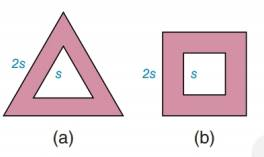Chapter 8.3, Problem 31EElementary Geometry For College St...

7th Edition
Alexander + 2 others
ISBN: 9781337614085

Solutions

Chapter
SectionElementary Geometry For College St...

7th Edition
Alexander + 2 others
ISBN: 9781337614085
Textbook Problem

Find the shaded area if thea) triangles are equilateralb) figures are squaresTo determine

a)

To find:

The area of the shaded portion.

Explanation

The area of the equilateral triangle is given by, A=34s2 where s is side of the equilateral triangle.

Calculation:

It is given that the sides of the larger and smaller equilateral triangle are 2s and s respectively.

The area of shaded portion is given by

Area of larger equilateral triangle – Area of smaller equilateral triangle

=34(2s)234(s)2=34(4s2)3

To determine

b)

To find:

The area of the shaded portion.

Still sussing out bartleby?

Check out a sample textbook solution.

See a sample solution

The Solution to Your Study Problems

Bartleby provides explanations to thousands of textbook problems written by our experts, many with advanced degrees!

Get Started